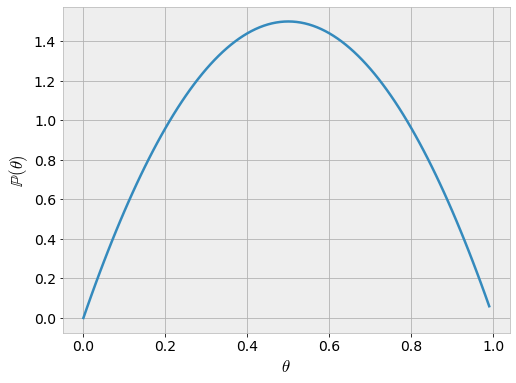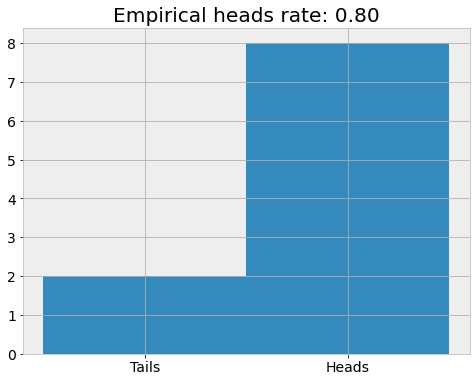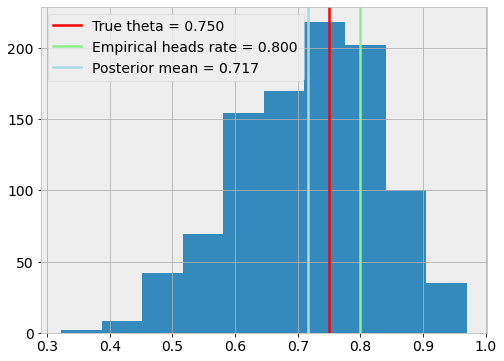# Coin flipping

## Tutorial: Estimating heads rate of a coin​

This tutorial demonstrates modeling and running inference on a simple coin-flipping model in Bean Machine. This should offer an accessible introduction to fundamental features of Bean Machine.

## Problem​

In this tutorial, we consider the problem of estimating the probability of heads for a potentially biased coin. In this setup, we have flipped the coin a few times and recorded the results as training data. We're interested in better understanding the coin's true heads rate.

## Prerequisites​

Let's code this in Bean Machine! Import the Bean Machine library and some fundamental PyTorch classes.

# Install Bean Machine in Colab if using Colab.import sysif "google.colab" in sys.modules and "beanmachine" not in sys.modules:    !pip install beanmachine
import osimport arviz as azimport beanmachine.ppl as bmimport beanmachine.ppl.inference.monte_carlo_samplesimport matplotlib.pyplot as pltimport pandas as pdimport torchimport torch.distributions as distfrom beanmachine.tutorials.utils import plotsfrom bokeh.io import output_notebookfrom bokeh.models import ColumnDataSourcefrom bokeh.palettes import Colorblind3from bokeh.plotting import gridplot, showfrom IPython.display import Markdownfrom torch import tensor

The next cell includes convenient configuration settings to improve the notebook presentation as well as setting a manual seed for reproducibility.

# Plotting settingsplt.rc("figure", figsize=[8, 6])plt.rc("font", size=14)plt.rc("lines", linewidth=2.5)# Manual seedbm.seed(11)torch.manual_seed(11)# Other settings for the notebook.smoke_test = "SANDCASTLE_NEXUS" in os.environ or "CI" in os.environ# Plotting settingsaz.rcParams["plot.backend"] = "bokeh"az.rcParams["stats.hdi_prob"] = 0.89

## Model​

We have the following definitions.

• $\theta \in [0, 1]$: Heads rate of the coin.
• $y_i \in \{0, 1\}$: Result of the $i$th coin flip. This is usually an observed value.
• $N \in \mathbb{Z}^+$: Number of observed coin flips.

We're interested in modeling:

• $\mathbb{P}(\theta | \{y_i\}_{i=1}^N)$

In Bayesian modeling, we encode our initial understanding of $\theta$ by specifying a prior distribution for it $\mathbb{P}(\theta)$. We then describe the relationship between the unobserved random variable $\theta$, and the observed random variables $\{y_i\}_{i=1}^N$. We can do this as follows:

• $\theta \sim \text{Beta}(2, 2)$
• $y_i \stackrel{iid}{\sim} \text{Bernoulli}(\theta)$

These will later combine to form the posterior distribution $\mathbb{P}(\theta | \{y_i\}_{i=1}^N)$ which encodes our improved understanding of $\theta$.

As a convention, we will consider an outcome of 1 from the Bernoulli distribution to represent heads and zero to represent tails. Here we chose to use a Beta distribution for the prior distribution for the coin's probability of heads, but in principle any distribution over $[0, 1]$ can be used.

Let's visualize the particular Beta distribution used in this tutorial:

thetas = torch.arange(0, 1, 0.01)plt.plot(thetas, dist.Beta(2, 2).log_prob(thetas).exp())plt.xlabel(r"$\theta$")plt.ylabel(r"$\mathbb{P}(\theta)$");We can implement this model in Bean Machine by defining random variable objects with the @bm.random_variable decorator. These functions behave differently than ordinary Python functions.

Semantics for @bm.random_variable functions:
• They must return PyTorch Distribution objects.
• Though they return distributions, callees actually receive a sample from the distribution. The machinery for obtaining samples from distributions is handled internally by Bean Machine.
• Inference runs the model through many iterations. During a particular inference iteration, a distinct random variable will correspond to exactly one sampled value: calls to the same random variable function with the same arguments will receive the same sampled value within one inference iteration. This makes it easy for multiple components of your model to refer to the same logical random variable.
• Consequently, to define distinct random variables that correspond to different sampled values during a particular inference iteration, an effective practice is to add a dummy "indexing" parameter to the function. Distinct random variables can be referred to with different values for this index.

Semantics for @bm.random_variable functions:

• This is a decorator that let's you treat deterministic code as if it's a Bean Machine random variable. This is used to transform the results of one or more random variables.
• This follows the same naming practice as @bm.random_variable where variables are distinguished by their argument call values.

First, let's define our prior for the heads rate, $\theta$, using this syntax:

@bm.random_variabledef theta():    return dist.Beta(2, 2)

Similarly, let's define a random variables for the observed values, $y_i$:

@bm.random_variabledef y(i: int):    return dist.Bernoulli(theta())

The mathematical definitions map directly to the Python definitions.

In contrast to theta(), notice that y(i) defines a dummy indexing parameter i. That's because y(i) is actually defining a family of random variables -- one for each observed coin flip! This allows us to refer to specific observations as y(0), y(1), and so on.

In the above examples, we've defined our random variable functions in the toplevel for convenience. It's worth noting that Bean Machine models can be packaged into Python classes and instantiated as needed. This essentially namespaces random variables defined within, since the self argument that each random variable receives will be associated with the distinct instantiated class that it's a part of. Example code is included as an illustration below; however, this tutorial will continue to use the toplevel definitions.

class HeadsRateModel(object):    @bm.random_variable    def theta(self):        return dist.Beta(2, 2)    @bm.random_variable    def y(self, i):        return dist.Bernoulli(self.theta())heads_rate_model = HeadsRateModel()

## Data​

With the model defined, we need to collect some observed data in order to learn about values of interest in our model.

In this case, we will observe a few coin flips. For demonstrative purposes, we will use a synthetically generated dataset of observed values. In practice, you would flip a real coin and record observations instead.

For our synthetic dataset, we will assume a true heads rate of $0.75$:

true_theta = 0.75true_y = dist.Bernoulli(true_theta)

Now, we'll gather $10$ observations from flipping that coin.

N = 10y_obs = true_y.sample((N,))
y_obs
Out:
tensor(1.)

Let's analyze the dataset:

plt.hist(y_obs.numpy(), bins=2)plt.xticks([0.25, 0.75], ["Tails", "Heads"])plt.title(f"Empirical heads rate: {y_obs.mean() :.2f}");## Inference​

Inference is the process of combining model and data to obtain insights, in the form of probability distributions over values of interest. Bean Machine offers a powerful and general inference framework to enable fitting a arbitrary models to data.

Our inference algorithms expect observations in the form of a dictionary. This dictionary should consist of @bm.random_variable invocations as keys, and tensor data as values. You can see this in the example below:

observations = {    y(0): y_obs,    y(1): y_obs,    y(2): y_obs,    y(3): y_obs,    y(4): y_obs,    y(5): y_obs,    y(6): y_obs,    y(7): y_obs,    y(8): y_obs,    y(9): y_obs,}

Now, we're ready to run inference! For this very simple tutorial, we'll use the simple Metropolis-Hastings inference algorithm. Check out the documentation or other tutorials for more advanced examples!

Running inference consists of a few arguments:

NameUsage
queriesList of @bm.random_variable targets to fit posterior distributions for.
observationsA dictionary of observations.
num_samplesNumber of Monte Carlo samples to approximate the posterior distributions for the variables in queries.
num_chainsNumber of separate inference runs to use. Multiple chains can help verify that inference ran correctly.

Let's run inference:

num_samples = 2 if smoke_test else 1000samples = bm.SingleSiteAncestralMetropolisHastings().infer(    queries=[theta()],    observations=observations,    num_samples=num_samples,    num_chains=4,)
Out:
Samples collected:   0%|          | 0/1000 [00:00<?, ?it/s]Samples collected:   0%|          | 0/1000 [00:00<?, ?it/s]Samples collected:   0%|          | 0/1000 [00:00<?, ?it/s]Samples collected:   0%|          | 0/1000 [00:00<?, ?it/s]

## Analysis​

samples now contains our inference results. Although we ran inference only to compute distributions for theta(), in general you can compute distributions for many variables at once. So, you have to index into this object using the random variable syntax we've seen several times. Here's an example:

theta_for_four_chains = samples[theta()].detach()theta_for_four_chains
Out:
tensor([[0.7710, 0.7710, 0.7710,  ..., 0.8267, 0.8267, 0.8086],        [0.2854, 0.2854, 0.7474,  ..., 0.7243, 0.7243, 0.7865],        [0.8248, 0.7088, 0.7088,  ..., 0.7886, 0.7886, 0.7886],        [0.6676, 0.7408, 0.6948,  ..., 0.7648, 0.7648, 0.7648]])

Results from all four separate inference runs are stored in here. We'll use them later; for now, let's just examine one of these runs:

theta_for_first_chain = theta_for_four_chainsplt.hist(theta_for_first_chain.numpy())plt.axvline(true_theta, color="red", label=f"True theta = {true_theta :.03f}")plt.axvline(    y_obs.mean(),    color="lightgreen",    label=f"Empirical heads rate = {y_obs.mean() :.03f}",)plt.axvline(    theta_for_first_chain.mean(),    color="lightblue",    label=f"Posterior mean = {theta_for_first_chain.mean() :.03f}",)plt.legend();The graph above is based on pseudo-randomly generated data, so, the results can be varied by changing the seed. Typically,

<!--

the true $\theta$ will remain within the main non-zero probability part of the histogram, but may not necessarily fall in the modal interval of the histogram. More generally, for this particular problem, we can expect --> the mean of inferred distribution will be closer to 0.5 than the true $\theta$. This is because 0.5 is the mean of our prior for $\theta$.

Arviz provides helpful statistics about the result of the inference algorithm. We can query this information as follows:

Markdown(az.summary(samples.to_inference_data(), round_to=4).to_markdown())
meansdhdi_5.5%hdi_94.5%mcse_meanmcse_sdess_bulkess_tailr_hat
theta()0.71610.11540.55270.90350.00360.00251051.7914.0831.006

As is suggested by the visualization, the average and the median are around $0.72$. Moreover, 95% of the samples fall within the interval $[0.460, 0.904]$. The width of this interval reflects the reality that we have conditions on few observations, and so should be quite uncertain about the true $\theta$.

As is suggested by the visualization, the average and the median are around $0.72$. Moreover, 95% of the samples fall within the interval $[0.460, 0.904]$. The width of this interval reflects the reality that we have conditions on few observations, and so should be quite uncertain about the true $\theta$.

The summary output shows two diagnostic statistics: $\hat{R}$ (r_hat) and $N_\text{eff}$ (effective sample size, n_eff).

• $\hat{R} \in [1, \infty)$ summarizes how effective inference was at converging on the correct posterior distribution for a particular random variable. It uses information from all chains run in order to assess whether inference had a good understanding of the distribution or not. Values very close to zero indicate that all chains discovered similar distributions for a particular random variable. We do not recommend using inference results where $\hat{R} > 1.1$, as inference may not have converged. In that case, you may want to run inference for more samples.
• $N_\text{eff} \in [1, \texttt{num}\_\texttt{samples}]$ summarizes how independent posterior samples are from one another. Although inference was run for num_samples iterations, it's possible that those samples were very similar to each other (due to the way inference is implemented), and may not each be representative of the full posterior space. Larger numbers are better here, and if your particular use case calls for a certain number of samples to be considered, you should ensure that $N_\text{eff}$ is at least that large.

In this case, $\hat{R}$ and $N_\text{eff}$ seem to have acceptable values.

Bean Machine can also plot diagnostical information to assess model fit. Let's take a look:

# Required for visualizing in Colab.output_notebook(hide_banner=True)samples_diagnostic_plots = gridplot(plots.plot_diagnostics(samples))show(samples_diagnostic_plots)

The diagnostics output shows two diagnostic plots for individual random variables: trace plots and autocorrelation plots.

• Trace plots are simply a time series of values assigned to random variables over each iteration of inference. The concrete values assigned are usually problem-specific. However, it's important that these values are "mixing" well over time. This means that they don't tend to get stuck in one region for large periods of time, and that each of the chains ends up exploring the same space as the other chains throughout the course of inference.
• Autocorrelation plots measure how predictive the last several samples are of the current sample. Autocorrelation may vary between -1.0 (deterministically anticorrelated) and 1.0 (deterministically correlated). (We compute autocorrelation approximately, so it may sometimes exceed these bounds.) In an ideal world, the current sample is chosen independently of the previous samples: an autocorrelation of zero. This is not possible in practice, due to stochastic noise and the mechanics of how inference works.

From the trace plots, we see each of the chains are healthy: they don't get stuck, and do not explore a chain-specific subset of the space. From the autocorrelation plots, we see the absolute magnitude of autocorrelation to be very small, often around 0.1, indicating a healthy exploration of the space.

## BMGInference​

Bean Machine Graph (BMG) Inference is an experimental feature of the Bean Machine framework that aims to deliver higher performance for specialized models. The model used in this tutorial represents a static probabilistic graph model and happens to use only features within the language subset supported by BMGInference. Currently, however, only Newtonian Monte Carlo (NMC) inference is supported by BMGInference. So, as a reference point, the following code reports the time it takes for our basic implementation of NMC to compute the posterior:

%%timesamples = bm.SingleSiteNewtonianMonteCarlo().infer(    queries=[theta()],    observations=observations,    num_samples=num_samples,    num_chains=4,)
Out:
Samples collected:   0%|          | 0/1000 [00:00<?, ?it/s]Samples collected:   0%|          | 0/1000 [00:00<?, ?it/s]Samples collected:   0%|          | 0/1000 [00:00<?, ?it/s]Samples collected:   0%|          | 0/1000 [00:00<?, ?it/s]CPU times: user 10.7 s, sys: 68 ms, total: 10.8 sWall time: 10.8 s

To run our model using BMGInference, the only change needed is the following:

%%timefrom beanmachine.ppl.inference.bmg_inference import BMGInferencesamples_bmg = BMGInference().infer(    queries=[theta()],    observations=observations,    num_samples=num_samples,    num_chains=4,)
Out:
0%   10   20   30   40   50   60   70   80   90   100%|----|----|----|----|----|----|----|----|----|----|CPU times: user 1.5 s, sys: 11.8 ms, total: 1.51 sWall time: 1.51 s***************************************************

Wall time numbers will naturally vary on different platforms, but with with these parameters (model, observations, queries, sample size, and number of chains) speedup on the author's machine is about 40x. Generally speaking, larger speedups are expected with larger sample sizes. More information about BMGInference can be found on the website in "Advanced" section of the documentation.